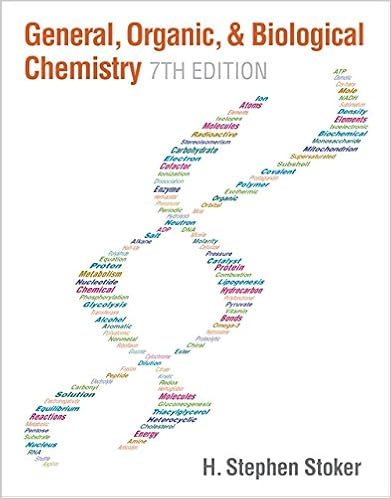# C 6 h 8 o 6aq i 2aq c 6 h 6 o 6aq 2h aq 2i aq and

• Notes
• 220
• 100% (1) 1 out of 1 people found this document helpful

This preview shows page 209 - 220 out of 220 pages.

##### We have textbook solutions for you!
The document you are viewing contains questions related to this textbook.The document you are viewing contains questions related to this textbook.
Chapter 15 / Exercise 15-83
General, Organic, & Biological Chemistry
StokerExpert Verified
C 6 H 8 O 6(aq) + I 2(aq) C 6 H 6 O 6(aq) + 2H + (aq) + 2I - (aq) And then to titrate the iodine that did not react with the ascorbic acid with sodium thiosulfate. The balanced, net ionic equation for the reaction occurring in this titration is I 2(aq) + 2 S 2 O 3 2- (aq) 2I - (aq) + S 4 O 6 2- (aq) Suppose 50.00 mL of 0.0520 M I 2 was added to the sample containing ascorbic acid. After the ascorbic acid/ I 2 reaction was complete, the I 2 not used in the reaction required 20.30 mL of 0.196 M sodium thiosulfate (Na 2 S 2 O 3 ) for titration to the equivalence point. Calculate the mass of ascorbic acid in the unknown sample.
##### We have textbook solutions for you!
The document you are viewing contains questions related to this textbook.The document you are viewing contains questions related to this textbook.
Chapter 15 / Exercise 15-83
General, Organic, & Biological Chemistry
StokerExpert Verified
The Equilibrium State All reactions are reversible and under suitable conditions will reach a state of equilibrium .
The Equilibrium State All reactions are reversible and under suitable conditions will reach a state of equilibrium . At equilibrium, the concentrations of products and reactants no longer change because the rates of the forward and reverse reactions are equal. Rates equal NOT the concentrations! At equilibrium: rate forward = rate reverse
The Equilibrium State All reactions are reversible and under suitable conditions will reach a state of equilibrium . At equilibrium, the concentrations of products and reactants no longer change because the rates of the forward and reverse reactions are equal. At equilibrium: rate forward = rate reverse Chemical equilibrium is a dynamic state because reactions continue to occur, but because they occur at the same rate, no net change is observed on the macroscopic level .
The Equilibrium Constant You will do this in Chapter 17 not now. At equilibrium rate fwd = rate rev so k [N 2 O 4 ] eq = k [NO 2 ] 2 eq k fwd k rev [NO 2 ] [N 2 O 4 ] eq 2 eq = then The ratio of constants gives a new constant, the equilibrium constant: k fwd k rev [NO 2 ] [N 2 O 4 ] eq 2 eq = K = Consider the reaction N 2 O 4 ( g ) 2NO 2 ( g )
K and the extent of reaction K reflects a particular ratio of product concentrations to reactant concentrations for a reaction. A small value for K indicates that the reaction yields little product before reaching equilibrium. The reaction favors the reactants . K therefore indicates the extent of a reaction, i.e., how far a reaction proceeds towards the products at a given temperature. A large value for K indicates that the reaction reaches equilibrium with very little reactant remaining. The reaction favors the products .
Figure 17.2 The range of equilibrium constants. small K The reaction mixture contains mostly reactants. The reaction barely goes forward. large K The reaction mixture contains mostly products. The reaction goes very far forward. intermediate K
Additional reading! Go to CH 14 and read page 529 and 532. Key atomic properties of Group 1A and 2A Atomic properties Physical properties Reactions
Ca 2+ + CO 3 2- CaCO 3 NH 3 + H + NH 4 + Zn + 2HCl ZnCl 2 + H 2 Ca + F 2 CaF 2 Classify the following reactions.
Ca 2+ + CO 3 2- CaCO 3 NH 3 + H + NH 4 + Zn + 2HCl ZnCl 2 + H 2 Ca + F 2 CaF 2 Precipitation Acid-Base Redox (H 2 Displacement) Redox (Combination) Classify the following reactions.
Oxidation-Reduction (Redox) Reactions Oxidation is the loss of electrons.
•••